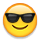P2 QDIV/QFRAC Clarification Needed — Parallax Forums

# P2 QDIV/QFRAC Clarification Needed

edited 2020-01-12 20:42
I must be misunderstanding how to use the cordic divider. I can find now way to finagle it to divide a 32-bit number into another 32-bit number in the manner needed for my solution.

In my use case, both dividend and divisor are left justified so that bit 31 of both is always set to 1. What differs between them is expressed in the remainder of the bits after bit 31, and also in the two exponent registers that hold the scaling factor for each.

Lets take a simple example without reference to scaling:

A = 0x80000000
B = 0x80000000

X = A/B

The result I need for my solution is:

X=0x80000000
Remainder=0x00000000

What I get instead is:
X=0x00000001
Remainder=0x00000000

The code for this is somewhat like:

QDIV A,B
GETQX X
GETQY Remainder

I also tried QFRAC, with no luck.

My current bit-banging code works well but consumes a huge number of cycles. I will try to post that next, if I can figure out how to format it so it's not all squished up against the left column.

(Edited for corrections)

## Comments

• Here is the bit-banging code I currently use to divide the way I need.
```_Div            mov     temp1,frac64Ah
mov     temp2,#0
mov     frac64Ah,#0
sub     k_expA,k_expB

' set the loop counter
mov     cntr1,#32

_A1             sub     temp2,frac64Bl  wc,wz
subx    temp1,frac64Bh  wc,wz

' toggle the carry flag for later testing
muxnc   tmpx,bit_31
if_ae   shl     temp2,#1    wc
if_ae   rcl     temp1,#1
if_ae   jmp     #_A2

' add back the divisor
add     temp2,frac64Bl  wc
addx    temp1,frac64Bh
shr     frac64Bh,#1     wc
rcr     frac64Bl,#1

_A2             mov     tmpx,tmpx       wc
rcl     frac64Ah,#1     wc
djnz    cntr1,#_A1

test    frac64Ah,bit_31 wz
if_nz   jmp     #__pack_results
sub     k_expA,#1
shl     frac64Al,#1     wc
rcl     frac64Ah,#1

jmp     #__pack_results

k_expA          long    0
frac64Al        long    0
frac64Ah        long    0

k_expB          long    0
frac64Bh        long    0
frac64Bl        long    0

temp1           long    2
temp2           long    3
```
• QFRAC A,B will work like you want if you shift A right by one bit.
• > @MSwann said:
> I must be misunderstanding how to use the cordic divider. I can find now way to finagle it to divide a 32-bit number into another 32-bit number in the manner needed for my solution.
>
> In my use case, both dividend and divisor are left justified so that bit 31 of both is always set to 1. What differs between them is expressed in the remainder of the bits after bit 31, and also in the two exponent registers that hold the scaling factor for each.
>
> Lets take a simple example without reference to scaling:
>
> A = 0x80000000
> B = 0x80000000
>
> X = A/B
>
> The result I need for my solution is:
>
> X=0x80000000
> Remainder=0x00000000
>
> What I get instead is:
> X=0x00000001
> Remainder=0x00000000
>
> The code for this is somewhat like:
>
> QDIV A,B
> GETQX X
> GETQY Remainder
>
> I also tried QFRAC, with no luck.
>
> My current bit-banging code works well but consumes a huge number of cycles. I will try to post that next, if I can figure out how to format it so it's not all squished up against the left column.
>
> (Edited for corrections)

80000000 / 80000000 = 00000001 r=00000000
• I find it's always helpful in this kind of situation to figure out exactly what's going on, mathematically. You want the result A/B as a 1.31 fixed point number, but QDIV calculates it as a plain integer and remainder. Another way to think of it is that you have:

A = 2^31 * a (where a is a real number 0 <= a < 2
B = 2^31 * b (where b is a real number 0 <= b < 2

and you want to calculate

X = 2^31 * (a/b)

The hardware will calculate A/B exactly, but

A/B = (2^31*a) / (2^31*b) = a/b

whereas you actually want the result represented as a 1.31 fixed point number, i.e. 2^31 * (a/b).

There are two ways to fix this:

(1) Scale the result after the division. This is very prone to error; a/b itself is probably just 0 or 1, so you'd have to figure out based on b and the remainder what the rest of the bits should be.

(2) Scale A before division; mathematically 2^31 * (a / b) = 2^31 * (A /= (2^31 * A) / B. In this case you'll have to represent 2^31*A as a 64 bit number, but that's OK, the QDIV instruction can do 64 bit by 32 bit division.
```   mov  Xhi, A
mov  X, #0  ' now Xhi, X is A*2^32 as a 64 bit number
shr  Xhi, #1 wc
rorc X, #1  ' now Xhi,X is A*2^31 as a 64 bit number
setq Xhi
qdiv X, B  ' 64 bit division of Xhi,X / B
getqx X ' now X is (2*31 * A) / B
```
You can get fancy and check the remainder if you want to round X correctly.

If you happen to know that A/B is less than 1 (so A < B ) then you could simplify this quite a bit by just doing qfrac A,B and shifting the result right by 1, i.e. calculating 2^32*A / B and then dividing by 2 to get 2^31 * A / B. Or you could shift A right by 1 and calculate 2^31 * A/B == 2^32 * (A/2) / B. You'll lose a little bit of precision, but it's marginally faster. The big cost is the qfrac/qdiv anyway.
• Thanks @ersmith and @cgracey. That did the trick.
Sign In or Register to comment.